# DAV Class 4 Maths Chapter 11 Brain Teasers Solutions

The DAV Maths Book Class 4 Solutions and DAV Class 4 Maths Chapter 11 Brain Teasers Solutions of Perimeter offer comprehensive answers to textbook questions.

## DAV Class 4 Maths Ch 11 Brain Teasers Solutions

Question 1.
(a) Which of the following does not belong to the group?(b) Perimeter of a triangle is 15 cm. If length of one side is 4 cm, the sum of other two sides will be __________.
(i) 19 cm
(ii) 11 cm
(iii) 12 cm
(iv) 6 cm
Perimeter of triangle = 15 cm
one side = 4 cm
Sum of other two sides = Perimeter – One side
= 15 – 4
= 11 cm

(c) Rahul covered a distance of 800 m in running around a square garden once. The length of a side of the garden is __________.
(i) 400 m
(ii) 200 m
(in) 800 m
(iv) 100 m
Perimeter of garden = 800 m
Perimeter of square garden = 4 × side 800 m
= 4 × side
(800 ÷ 4) m = side
200 m = side

(d) I need __________ of ribbon to make a border around a rectangular picture of length 20 cm and breadth 8 cm?
(i) 28 cm
(ii) 56 cm
(iii) 48 cm
(iv) 36 cm
Perimeter of rectangular picture
= 2(l) + 2(b)
= 2 (20) + (8) × 2
= 56 cm

(e) Perimeter of the given figure is __________ cm.(i) 18 cm
(ii) 15 cm
(iii) 16 cm
(iv) 12 cm
Perimeter of given figure
= 4 + 2 + 3 + 3 + 1 + 3
= 16 cmQuestion 2.
Find the perimeter of the following: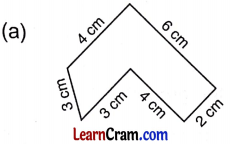Perimeter of figure
= 4 cm + 3 cm + 3 cm + 4 cm + 2 cm + 6 cm
= 22 cm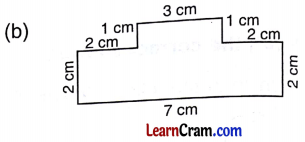Perimeter of figure
= 3 cm + 1 cm + 2 cm + 2 cm + 7 cm + 2 cm + 2 cm + 1 cm
= 20 cm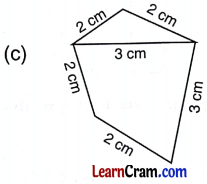Perimeter of figure
= 2 cm + 2 cm + 2 cm + 3 cm + 2 cm
= 11 cmPerimeter of figure
= 5 cm + 1 cm + 5 cm + 2cm + 2 cm
= 15 cm

Question 3.
Renu is having a rectangular field whose length is 7 m and breadth is 4 meters. Find the perimeter of the field.
Perimeter of rectangular field = 2l + 2 b
= 2 × 7m + 2 × 4m
= 14 m + 8 m
= 22 m
Perimeter of field = 22 m

Question 4.
Mr Kumar has a farmhouse. The shape of boundary of his farmhouse is given below: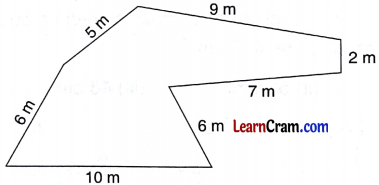Mr Kumar wants to fence his farmhouse with barbed wire. If the cost if fencing is ₹ 25 per metre
To find the amount spend on fencing we have to find perimeter of farmhouse.
Perimeter of farm house
= 10m + 6m + 7m + 2m + 9m + 5m + 6m
= 45 m

Per metre cost of fencing is ₹ 25
45 metre cost of fencing is ₹ 25 × 45 m
= ₹ 1,125
₹ 1,125 is cost of fencing.Question 5.
Find the perimeter of given figure.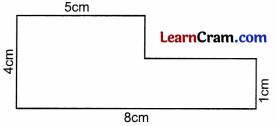Perimeter of given figure
= 4 cm + 5 cm + 3 cm + 3 cm + 1 cm + 8 cm
= 24 cm
Perimeter = 24 cm 5cm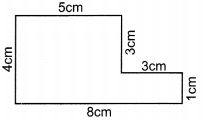Question 6.
My mother has 90 cm lace with her. She wants to stitch this lace on the border of my square handkerchief. If the length of each side of my handkerchief is 20 cm, find the length of the remaining lace.
Total length of lace = 90 cm
Length of each side of handkerchief = 20 cm
Perimeter of square handkerchief = 4 × side
= 4 × 20
= 80 cm
Length of remaining lace = 90 cm – 80 cm
= 10 cm

Question 1.
Classify as open figure and closed figure.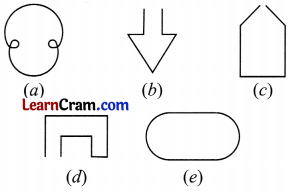Open figures – (b), (c), (d)
Closed figures – (a), (e)Question 2.
Find the perimeter of following figures.Perimeter of figure
= 6 cm + 5 cm + 1 cm + 2 cm + 2 cm + 2 cm + 1 cm + 5 cm
= 24 cmPerimeter of figure
= 2 cm + 3 cm + 4 cm + 3 cm + 2 cm
= 14 cm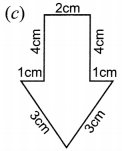Perimeter of figure
= 3 cm + 3 cm + 1 cm + 4 cm + 2cm + 4 cm + 1 cm
= 18 cmPerimeter of figure
= 5 + 5 + 2 + 4 + 4
= 20 cm

Question 3.
Formulate the perimeter of following figures:
(a) Rectangle
if length = a cm, breadth = b cm
Rectangle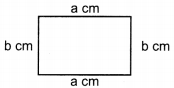Perimeter = a cm + b cm + a cm + b cm
= 2a cm + 2b cm

(b) Square
if side = x cm
Square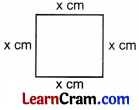Perimeter of square = sum of all sides
= x cm + x cm + x cm + x cm
= 4x cm

(c) Triangle
if sides are l cm, m cm, n cm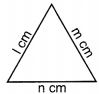= Sum of all sides
Perimeter = 1 cm + m cm + n cmQuestion 4.
Find the perimeter of a triangle two of whose sides are each 15 cm long and third side is 20 cm long.Perimeter of triangle = sum of three sides
= 15 cm + 15 cm + 20 cm
= 50 cm

Question 5.
The length of square field is 56 metres. What length of wire will be needed for fencing? Also find the cost of fencing if cost of per metre is ₹ 9.